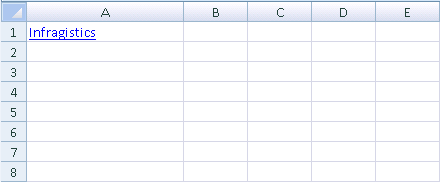Version

This topic shows you how to add a hyperlink to a cell in a Microsoft® Excel™ file using the WorksheetCell object’s ApplyFormula method. The following code creates an Excel file with a hyperlink in cell 0 of the worksheet by using the intrinsic Excel HYPERLINK formula.

Before you start writing any code, you should place using/imports directives in your code-behind so you don’t need to always type out a member’s fully qualified name.

In Visual Basic:

`Imports Infragistics.Documents.Excel`

In C#:

`using Infragistics.Documents.Excel;`

In Visual Basic:

```'Create an Excel File
Dim s As String = "C:\theFile.xls"
'Create a Workbook
Dim w As New Workbook()
'Add a new worksheet to the Workbook
Dim ws As Worksheet = w.Worksheets.Add("New")
'Create Hyperlink in a Worksheet cell
w.Save(s)```

In C#:

```//Create an Excel File
string s = @" C:\theFile.xls";
//Create a Workbook
Workbook w = new Workbook();
//Add a new worksheet to the Workbook